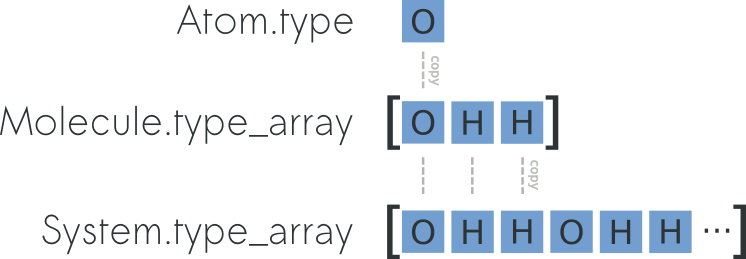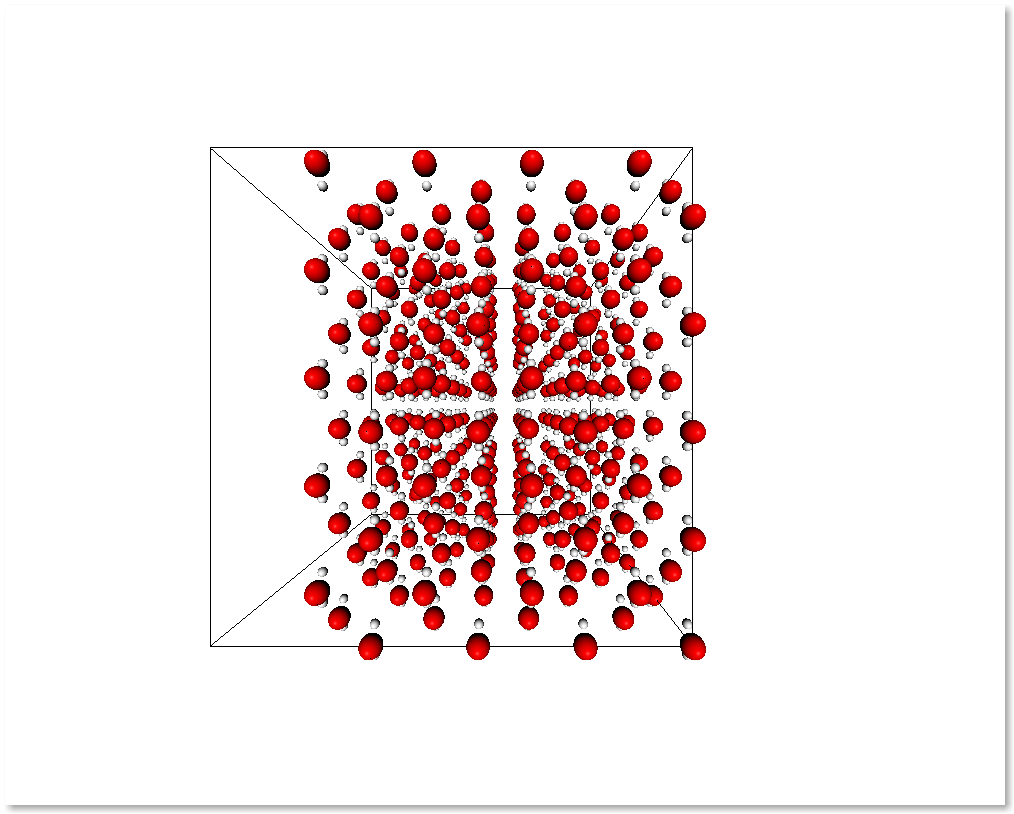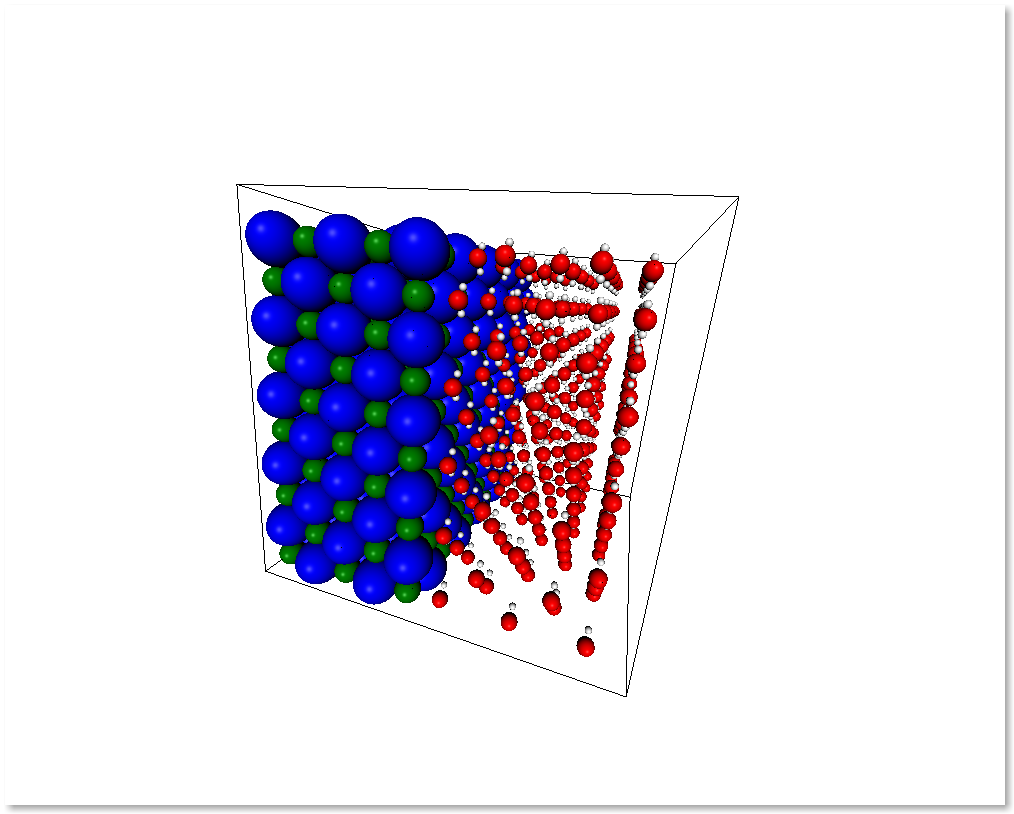# Atoms, Molecules and Systems¶

In chemlab, atoms can be represented using the chemlab.core.Atom data structure that contains some common information about our particles like type, mass and position. Atom instances are easily created by initializing them with data

>>> from chemlab.core import Atom
>>> ar = Atom('Ar', [0.0, 0.0, 0.0])
>>> ar.type
'Ar'
>>> ar.r
np.array([0.0, 0.0, 0.0])


Note

for the atomic coordinates you should use nanometers

A chemlab.core.Molecule is an entity composed of more atoms and most of the Molecule properties are inherited from the constituent atoms. To initialize a Molecule you can, for example pass a list of atom instances to its constructor:

>>> from chemlab.core import Molecule
>>> mol = Molecule([at1, at2, at3])


## Manipulating Molecules¶

Molecules are easily and efficiently manipulated through the use of numpy arrays. One of the most useful arrays contained in Molecule is the array of coordinates Molecule.r_array. The array of coordinates is a numpy array of shape (NA,3) where NA is the number of atoms in the molecule. According to the numpy broadcasting rules, if you sum two arrays with shapes (NA,3) and (3,), each row of the first array get summed by the second array. Let’s say we have a water molecule and we want to displace it randomly in a box, this is easily accomplished by initializing a Molecule at the origin and summing its coordinates by a random displacement:

import numpy as np

wat = Molecule([Atom("H", [0.0, 0.0, 0.0]),
Atom("H", [0.0, 1.0, 0.0]),
Atom("O", [0.0, 0.0, 1.0])])

# Shapes (NA, 3) and (3,)
wat.r_array += np.random.rand(3)


Using the same principles you can also apply other kinds of transformations such as matrices. You can for example rotate the molecule by 90 degrees around the z-axis:

from chemlab.graphics.transformations import rotation_matrix

# The transformation module returns 4x4 matrices
M = rotation_matrix(np.pi/2, np.array([0.0, 0.0, 1.0]))[:3,:3]

# slow, readable way
for i,r in enumerate(wat.r_array):
wat.r_array[i] = np.dot(M,r)

# numpy efficient way to do the same:
# wat.r_array = np.dot(wat.r_array, M.T)


The array-based API provides a massive increase in performance and a more straightforward integration with C libraries thanks to the numpy arrays. This feature comes at a cost: the data is copied between atoms and molecules, in other words the changes in the costituents atoms are not reflected in the Molecule and viceversa. Even if it may look a bit unnatural, this approach limits side effects making the code more predictable and easy to follow.

## Systems¶

In context such as molecular simulations it is customary to introduce a new data structure called System. A System represents a collection of molecules, and optionally (but recommended) you can pass also periodic box information:

>>> from chemlab.core import System
# molecule = a list of Molecule instances
>>> s = System(molecules, boxsize=2.0)


System do not take directly Atom instances as its constituents, therefore if you need to simulate a system made of single atoms (say, a box of liquid Ar) you need to wrap the atoms into a Molecule:

>>> ar = Atom('Ar', [0.0, 0.0, 0.0])
>>> mol = Molecule([ar])


System, similarly to Molecule can expose data by using arrays and it inherits atomic data from the constituent molecules. For instance, you can easily and efficiently access all the atomic coordinates by using the attribute System.r_array. To understand the relation between Atom.r, Molecule.r_array and System.r_array you can refer to the picture below:You can preallocate a System by using the classmethod System.empty (pretty much like you can preallocate numpy arrays with np.empty or np.zeros) and then add the molecules one by one:

import numpy as np
from chemlab.core import Atom, Molecule, System
from chemlab.graphics import display_system

# Template molecule
wat = Molecule([Atom('O', [0.00, 0.00, 0.01]),
Atom('H', [0.00, 0.08,-0.05]),
Atom('H', [0.00,-0.08,-0.05])])

# Initialize a system with four water molecules.
s = System.empty(4, 12) # 4 molecules, 12 atoms

for i in range(4):
wat.move_to(np.random.rand(3)) # randomly displace the water molecule
s.add(wat) # data gets copied each time

display_system(s)


Since the data is copied, the wat molecule act as a template so you can move it around and keep adding it to the System.

Preallocating and adding molecules is a pretty fast way to build a System, but the fastest way (in terms of processing time) is to build the system by passing ready-made arrays, this is done by using chemlab.core.System.from_arrays().

### Building Crystals¶

chemlab provides an handy way to build crystal structures from the atomic coordinates and the space group information. If you have the crystallographic data, you can easily build a crystal:

from chemlab.core import Atom, Molecule, crystal
from chemlab.graphics import display_system

# Molecule templates
na = Molecule([Atom('Na', [0.0, 0.0, 0.0])])
cl = Molecule([Atom('Cl', [0.0, 0.0, 0.0])])

s = crystal([[0.0, 0.0, 0.0], [0.5, 0.5, 0.5]], # Fractional Positions
[na, cl], # Molecules
225, # Space Group
cellpar = [.54, .54, .54, 90, 90, 90], # unit cell parameters
repetitions = [5, 5, 5]) # unit cell repetitions in each direction

display_system(s)


Note

If you’d like to implement a .cif file reader, you’re welcome! Drop a patch on github.

### Manipulating Systems¶

#### Selections¶

You can manipulate systems by using some simple but flexible functions. It is really easy to generate a system by selecting a part from a bigger system, this is implemented in the functions chemlab.core.subsystem_from_atoms() and chemlab.core.subsystem_from_molecules().

Those two functions take as first argument the original System, and as the second argument a selection. A selection is either a boolean array that is True when we want to select that element and False otherwise or an integer array containing the elements that we want to select. By using those two functions we can create subsystem by building those selections.

The following example shows an easy way to take the molecules that contain atoms in the region of space x > 0.5 by employing subsystem_from_atoms():

import numpy as np
from chemlab.core import crystal, Molecule, Atom, subsystem_from_atoms
from chemlab.graphics import display_system

# Template molecule
wat = Molecule([Atom('O', [0.00, 0.00, 0.01]),
Atom('H', [0.00, 0.08,-0.05]),
Atom('H', [0.00,-0.08,-0.05])])

s = crystal([[0.0, 0.0, 0.0]], [wat], 225,
cellpar = [.54, .54, .54, 90, 90, 90], # unit cell parameters
repetitions = [5, 5, 5]) # unit cell repetitions in each direction

selection = s.r_array[:, 0] > 0.5
sub_s = subsystem_from_atoms(s, selection)

display_system(sub_s)It is also possible to select a subsystem by selecting specific molecules, in the following example we select the first 10 water molecules by using subsystem_from_molecules():

from chemlab.core import subsystem_from_molecules

selection = np.array([0, 1, 2, 3, 4, 5, 6, 7, 8, 9])
sub_s = subsystem_from_molecules(s, selection)


Note

chemlab will provide other selection utilities in the future, if you have a specific request, file an issue on github

#### Merging systems¶

You can also create a system by merging two different systems. In the following example we will see how to make a NaCl/H2O interface by using chemlab.core.merge_systems():

import numpy as np
from chemlab.core import Atom, Molecule, crystal
from chemlab.core import subsystem_from_atoms, merge_systems
from chemlab.graphics import display_system

# Make water crystal
wat = Molecule([Atom('O', [0.00, 0.00, 0.01]),
Atom('H', [0.00, 0.08,-0.05]),
Atom('H', [0.00,-0.08,-0.05])])

water_crystal = crystal([[0.0, 0.0, 0.0]], [wat], 225,
cellpar = [.54, .54, .54, 90, 90, 90], # unit cell parameters
repetitions = [5, 5, 5]) # unit cell repetitions in each direction

# Make nacl crystal
na = Molecule([Atom('Na', [0.0, 0.0, 0.0])])
cl = Molecule([Atom('Cl', [0.0, 0.0, 0.0])])

nacl_crystal = crystal([[0.0, 0.0, 0.0], [0.5, 0.5, 0.5]], [na, cl], 225,
cellpar = [.54, .54, .54, 90, 90, 90],
repetitions = [5, 5, 5])

water_half = subsystem_from_atoms(water_crystal,
water_crystal.r_array[:,0] > 1.2)
nacl_half = subsystem_from_atoms(nacl_crystal,
nacl_crystal.r_array[:,0] < 1.2)

interface = merge_systems(water_half, nacl_half)
display_system(interface)At the present time, the merging will avoid overlapping by creating a bounding box around the two systems and removing the molecules of the first system that are inside the second system bounding box. In the future there will be more clever ways to handle this overlaps.

#### Sorting¶

If you use chemlab in conjunction with GROMACS, you may use the chemlab.core.System.sort() to sort the molecules according to their molecular formulas before exporting. The topology file expect to have a file with the same molecule type ordererd.